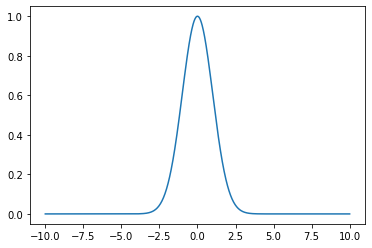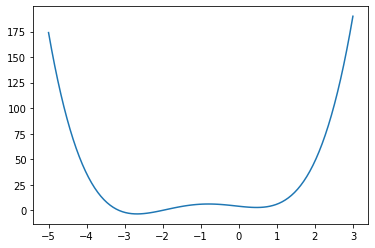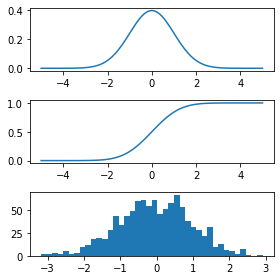# Lightspeed introduction to scipy¶

The scipy library is one of the core packages for scientific computing with Python.

It provides many user-friendly and efficient numerical routines such as routines for numerical integration and optimization.

Library documentation: http://www.scipy.org/

### Numerical integration¶

In :
from scipy.integrate import quad

x = np.linspace(-10, 10, 1000)
y = np.exp(- x ** 2 / 2)
_ = plt.plot(x, y)In :
# Integration
val, abserr = quad(lambda x: np.exp(-x ** 2 / 2),  -np.inf, np.inf)
val, (2 * np.pi) ** 0.5, abserr,

Out:
(2.5066282746309994, 2.5066282746310002, 2.5512942192316024e-08)

### Optimization¶

In :
def f(x):
return 4*x**3 + (x-2)**2 + x**4

x = np.linspace(-5, 3, 100)
_ = plt.plot(x, f(x))In :
import scipy as sp

x_min = sp.optimize.fmin_bfgs(f, -2)
print(x_min)

Optimization terminated successfully.
Current function value: -3.506641
Iterations: 5
Function evaluations: 24
[-2.67298151]

In :
x_min = sp.optimize.fmin_bfgs(f, 3)
print(x_min)

Optimization terminated successfully.
Current function value: 2.804988
Iterations: 9
Function evaluations: 30
[0.46961746]


### Statistics¶

In :
from scipy import stats

Y = stats.norm()
x = np.linspace(-5, 5, 100)
plt.figure(figsize=(4, 4))
plt.subplot(3, 1, 1)
plt.plot(x, Y.pdf(x)) # PDF function
plt.subplot(3, 1, 2)
plt.plot(x, Y.cdf(x)) # CDF function
plt.subplot(3, 1, 3)
_ = plt.hist(Y.rvs(size=1000), bins=40) # histogram of simulations
plt.tight_layout()In :
Y.mean(), Y.std(), Y.var()

Out:
(0.0, 1.0, 1.0)
In :
# t-test example
t_statistic, p_value = stats.ttest_ind(Y.rvs(size=1000), Y.rvs(size=1000))
t_statistic, p_value

Out:
(-0.21278093991656938, 0.8315195374950647)

### Exercice. MLE of a Gamma distribution¶

Let us recall that the density of a $\Gamma(a, \lambda)$ (Gamma distribution with shape parameter $a > 0$ and intensity $\lambda > 0$ is given by

$$f_{a, \lambda}(x) = \frac{\lambda^a}{\Gamma(a)} x^{a - 1} e^{-\lambda x} \mathbf 1_{x \geq 0}$$
1. Look for this distribution in scipy and plot its density function with shape=4.2 and intensity=1.5
2. Simulate a i.i.d sample of size $n=2000$ with this distribution
3. Write a function which computes the negative log-likelihood of this samples with prototype neg_log_lik(params) where params is an array of shape (2,) containing the scale and intensity
4. Use scipy.optim to minimize this function. Check that your estimator seems correct. Try to be clever with the initialization